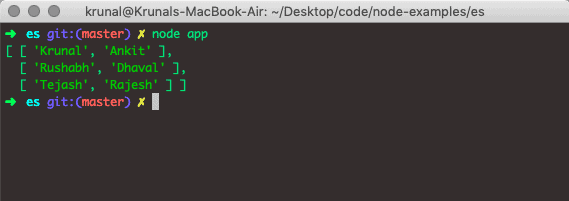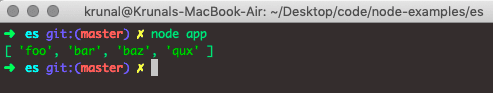# JavaScript array push: How to Add Element in Array

JavaScript array is a list object whose prototype has methods to perform the mutation operations. Neither a JavaScript array’s length nor its elements’ types are fixed.

Arrays are used to store multiple values in a single variable. An array data structure can hold many values under a single name, and you can access those values by referring to the index number.

## JavaScript array push

The array push() is a built-in JavaScript function that adds a new element at the end of an array and returns the new length. The array push() method accepts an element and appends it to the array.

The push() method modifies the length of the Array or collection and can append a number, string, object, array, or any value to the array.

### Syntax

`array.push(element)`

You can use a push() method to append more than one value to an array in a single call.

```array.push(item1, item2, item3,...,itemN)
```

### Parameters

The push() method takes the item(s) to add to the Array.

### Return Value

It returns the number representing the new length of the Array.

The push() method can be used with a call() or apply() on objects resembling the arrays.

The array.push()  method relies on the length property to resolve where to insert the given elements.

If the length cannot be converted into a number, the index used is 0.

It includes the possibility of length being nonexistent, in which case the length will also be created.

### Example

```data = ['Python', 'Javascript', 'PHP']
len = data.push('Golang')
console.log('The modified array is: ', data)
console.log('The length of modified array is: ', len)```

#### Output

```The modified array is:  [ 'Python', 'Javascript', 'PHP', 'Golang' ]
The length of modified array is:  4```

First, we defined an array, added a new item called Golang, and stored the new Array length. Then print the mutated Array and its length.

## Javascript add to an array.

Adding elements in the JavaScript array means appending as many elements as possible, like integers, strings, arrays, objects, an array of strings, an array of integers, and an array of arrays.

### Example

Let us take the example of Node.js to understand how to add an item in an array in JavaScript.

```// app.js

const songs = ['Dangerous', 'Thriller'];
const totalSongs = songs.push('Stranger In Moscow');

console.log(totalSongs);

console.log(songs);```

See the output.

```➜  es git:(master) ✗ node app
3
[ 'Dangerous', 'Thriller', 'Stranger In Moscow' ]
```

In this example, we added the third element, “Stanger in Moscow“. Then, after adding an item to the Array, we printed the mutated Array and its length.

## Adding multiple elements in the Array

To add multiple elements in the JavaScript array, use the array.push() function. We can add multiple elements as arguments at a single time.

### Example

```data = ['Python', 'Javascript', 'PHP']
len = data.push('Golang', 'C#', 'Swift')
console.log('The modified array is: ', data)
console.log('The length of modified array is: ', len)```

#### Output

```The modified array is:  [ 'Python', 'Javascript', 'PHP', 'Golang', 'C#', 'Swift' ]
The length of modified array is:  6```

We have added three elements in a single time, and all the elements are added in the Array.

The push() function can be called with multiple arguments, which will be appended to the array in order.

## Merging Arrays in Javascript using array push()

To merge arrays in JavaScript, use the array.push() function.

Let us take an example of how we can merge two arrays.

### Example

```// server.js

const artists = ['michael', 'elvis'];
const newArtists = ['justin', 'charlie'];

Array.prototype.push.apply(artists, newArtists);

console.log(artists);```

See the output.

```➜  es git:(master) ✗ node app
[ 'michael', 'elvis', 'justin', 'charlie' ]
➜  es git:(master) ✗
```

Do not use the apply() method if the second Array (newArtists in the example).

The maximum number of arguments one function can handle is limited in practice.

So, apply() will add the second Array to the first Array, and we can see the combined Array by returning the original Array, and in our case, it is artists.

To append the items of one Array to another array, you can use the concat() function of the Array. We can also use the JavaScript array concat() method to merge the arrays.

```// server.js

const artists = ['michael', 'elvis'];

artists.push('justin', 'charlie');

console.log(artists); // ['michael', 'elvis', 'justin', 'charlie']```

Here, we added two more artists.

```// server.js

const artists = ['michael', 'elvis'];
const newArtists = ['justin', 'charlie'];
artists.push(newArtists);

console.log(artists);```

See the output.

```➜  es git:(master) ✗ node app
[ 'michael', 'elvis', [ 'justin', 'charlie' ] ]
➜  es git:(master) ✗
```

Here, the third element is an array. So it has added the third item as a whole array. So we can not combine it as we have done it with apply().

We can remove the element from an array using the array pop() method.

## Adding Object to Array using array push()

To add an object to an array in JavaScript, use the array.push() method. The push() method adds one or multiple elements to an array’s end. The array.push() function returns the new length of the Array formed.

An object can be inserted, bypassing the object as a parameter to the push() method.

The object is hence added to the end of the Array.

### Example

Here, See the following code.

```// app.js

arrObj = [
{
name: 'Krunal',
age: 26
},
{
name: 'Ankit',
age: 24
}
];
arrObj.push({
name: 'Rushabh',
age: 27
});
console.log(arrObj);```

See the following output.

```➜  es git:(master) ✗ node app
[ { name: 'Krunal', age: 26 },
{ name: 'Ankit', age: 24 },
{ name: 'Rushabh', age: 27 } ]
➜  es git:(master) ✗
```

To add an object to the array, you must pass an object as an argument and ensure that the keys are the same. So, this is How to push keys and values into an Array in Javascript.

## Adding Array into the Array using push()

To add an array into an array in JavaScript, use the array.push() method. The push() function allows us to push an array into an array. We can add an array into an array, just like adding an element into the Array.

### Example

```// app.js

arrArray = [
['Krunal', 'Ankit'],
['Rushabh', 'Dhaval']
];
arrArray.push([
'Tejash', 'Rajesh'
]);
console.log(arrArray);```

See the output.If you’re only appending the single variable, then an array.push() method works like a charm.

If you have to append another array, use the concat() method.

This approach is beneficial when you don’t have to modify the original array, which is why it is standard practice and adapted by the Javascript community.

```data = ['Python', 'Javascript', 'PHP']
data2 = ['Java', 'Kotlin', 'Swift']

langs = data.concat(data2)
console.log(data)
console.log(data2)
console.log(langs)```

#### Output

```[ 'Python', 'Javascript', 'PHP' ]
[ 'Java', 'Kotlin', 'Swift' ]
[ 'Python', 'Javascript', 'PHP', 'Java', 'Kotlin', 'Swift' ]```

Javascript array concat() is a pure function and does not mutate its original array. Instead, it returns a new Array. So please ensure that the concat() function creates a new array.

It does not add or append to an existing instead, It simply creates a copy, performs the operation in that copy, and returns that array.

Javascript array.push.apply() function is also helpful to add an array into the array.

```let arr = ['Python'];
arr.push('Javascript', 'PHP');
arr.push.apply(arr, ['Dart', 'Golang']);
console.log(arr);```

#### Output

`[ 'Python', 'Javascript', 'PHP', 'Dart', 'Golang' ]`

You can use the push() and apply() functions to append two arrays.

Now, from the 2016 update: with spread, you don’t need that apply() method anymore.

```let arr = ['Python'];
arr.push('Javascript', 'PHP');
arr.push(...['Dart', 'Golang']);
console.log(arr);```

#### Output

`[ 'Python', 'Javascript', 'PHP', 'Dart', 'Golang' ]`

You can see that output will be the same, but this way is more concise and appealing to other coders.

## Appending multi-dimensional array in JavaScript

To append an element to a multi-dimensional array, use the array.push() method.

### Example

```// app.js
mulArray = [];
\$data = ['Tejash', 'Rajesh'];
\$info = [35, 40];
mulArray.push(\$data, \$info);
console.log(mulArray);
```

See the output.

```➜  es git:(master) ✗ node app
[ [ 'Tejash', 'Rajesh' ], [ 35, 40 ] ]
➜  es git:(master) ✗
```

There are several methods for appending new elements to the JavaScript array. If you instead want to remove elements from an array in JavaScript, we’ve got an article for that too.

## Adding an element to a specific position in the Array

To add an element to a particular position, you need to refer to its index position within the array. Here we add two new elements at specified positions.

### Example

```// app.js
let list = ["foo", "bar"];
list = "baz";
list = "qux";
console.log(list)
```

See the following output.## Adding an element at the beginning of the Array

Use unshift() method. It’s like push, except it inserts elements to the beginning of the Array instead of the end.

1. unshift/push: add an element to the beginning/end of an array.
2. shift/pop: remove and return the first/last element of an array.

A simple diagram is followed,

``````   unshift -> array <- push
shift   <- array -> pop``````

and the chart is the following.

``````          add  remove  start  end
push    X                   X
pop           X            X
unshift    X             X
shift           X      X``````

## Conclusion

JavaScript array push() is a function used to add one or more elements to an array’s end and returns the array’s new length. After the push() function is applied to an array, it will deliver a particular return value.

Suppose you have an array of the children of House Stark from Game of Thrones. However, one of the members, Bran, is missing, which is funny because he is the King of Six kingdoms.

Using the push() method, you could add him by assigning ‘Bran‘ to the array at the index after the last index.

That is it for this tutorial.

### 1 thought on “JavaScript array push: How to Add Element in Array”

1.thanks for the post